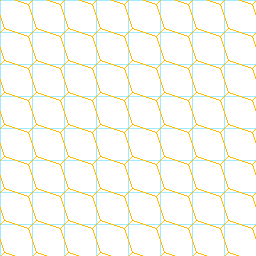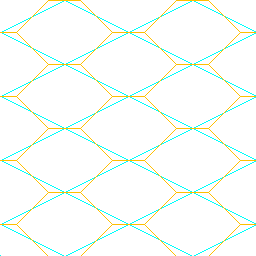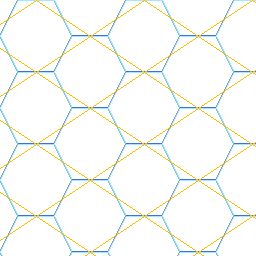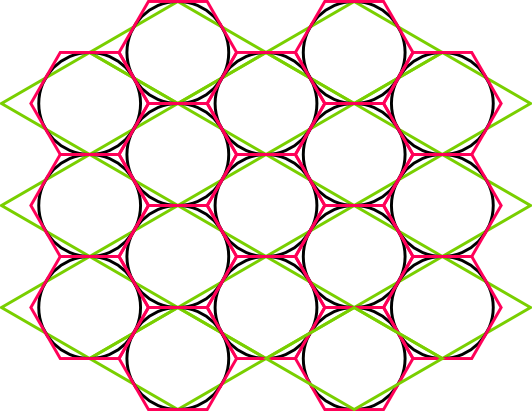# Introduction

Now that we know a bit about isometric maps - what about hexagon maps? Wikipedia only says this: http://en.wikipedia.org/wiki/Hex_map And this: http://en.wikipedia.org/wiki/Hexagon In games where neighbor tiles play a big role, hexagons are often preferable to the diamond/square tiles we looked at before. With diamonds/squares there are 4 neighbors with a common edge and 4 more neighbors with only a common vertex. With hexagons there are 6 neighbors and all equally share a common edge.

# Hexagons really are just diamonds/squares with corners cut away

First, realize that every hexagon map internally can just be seen as diamond/square map. Here are some examples. First we draw hexagons into a perfect square grid:Then in our nice 2:1 tiles isometric grid:And finally we try to put a grid over an existing hexagon map:As you can see each grid also is a hexmap and the other way around. And even more, each square/diamond corresponds to exactly one hexagon. So it will be extremely easy later handling hexmaps. We don't need to do anything special except find a convention which cells are neighbors and which not.

# The perfect hexagon

One interesting question now would be, what sort of grid do we get for a perfect hexagon map? Well, let's calculate it. In a perfect hexagon, each side has the same length and each angle is the same. So it gets clear that the length of each side s will be just the same as the length from the mid-point to a vertex. That means we simply put together three equal-sided triangles which have an angle of 60°. Therefore all the inner angles of our perfect hexagon are 120°. Pythagoras tells us that the distance from the center to the middle of a side is sqr(3)/2 which is about 0.866. The position of the 6 corners of our hexagon, if we start to the left, would therefore be: (-s, 0), (-0.5 s, 0.866 s), (0.5 s, 0.866 s), (s, 0), (0.5 s, -0.866 s), (-0.5 s, -0.866 s) And the vector from the hexagon center to the neighbor right up: (1.5 s, 0.866 s) And here's how it looks:One thing gets obvious when working with perfect hexagons - since there's always that 0.866 factor and in classic pixel drawing applications, like in the Gimp, you get only an integer grid, it's not very practical. Of course just using an almost perfect hexagon, but rounded to integer, solves this problem. Or using a vector application like Inkscape, which was used for the above picture.

# Pixel artist's and modeler's perfect hexagon

Now, above, we have seen different hexagons and there are many more. To find the best one to actually use for a map we could do something like with our iso map. Specify that we want perfect hexagons when looking at our map from directly above and then just view them from an angle to get some other wanted effect. Another idea would be to use the exact same projection as for our 2:1 iso tiles (so e.g. unit artwork could be re-used :) ). As seen in the second picture, this could be used to nicely overlay a hexagon map over our iso map. The real, unprojected hexagons would not be real hexagons but it wouldn't matter. And of course, also something like the 3rd picture works very well. This simply draws the hexagon into a 4x4 square grid, fitting each hexagon exactly into a square. It's what makes the pixel artists happy :) This again is not a projection of a perfect hexagon map. If a 3d modeler with perfect hexagons is used then grid settings can be used to get the 0.866 factor in there. Just make the grid 0.866 in one direction and 1 in the other. Hexagons fit in then with grid snapping. Maybe an interesting question is, in a 3D modeler, what would be the perspective to get a square grid to look like the diamond grid for our perfect hexes? We found out that the ratio of height to width is 0.866 / 1.5 - so just in the same way we did it with our 2:1 isometric tiles, we know that the view angle is asin(0.866 / 1.5) or asin(sqrt(3) / 3) or about 35.264 degree. That's how much we tilt our view down from viewing perfectly horizontal. (Viewing from directly above, asin(1/1), would be 90°, viewing horizontal, asin (0/1), would be 0°.) If you remember, with our 2:1 iso tiles we got asin(1/2) which was exactly 30°. So, the difference is not very big.. unit art could still be used. With the hexes in the 3rd picture we would get asin(2/3) which is about 41.810 degree. These values could maybe be used for artwork to use on the hex map if no real perfect 3d hexes are actually used in the modeler.